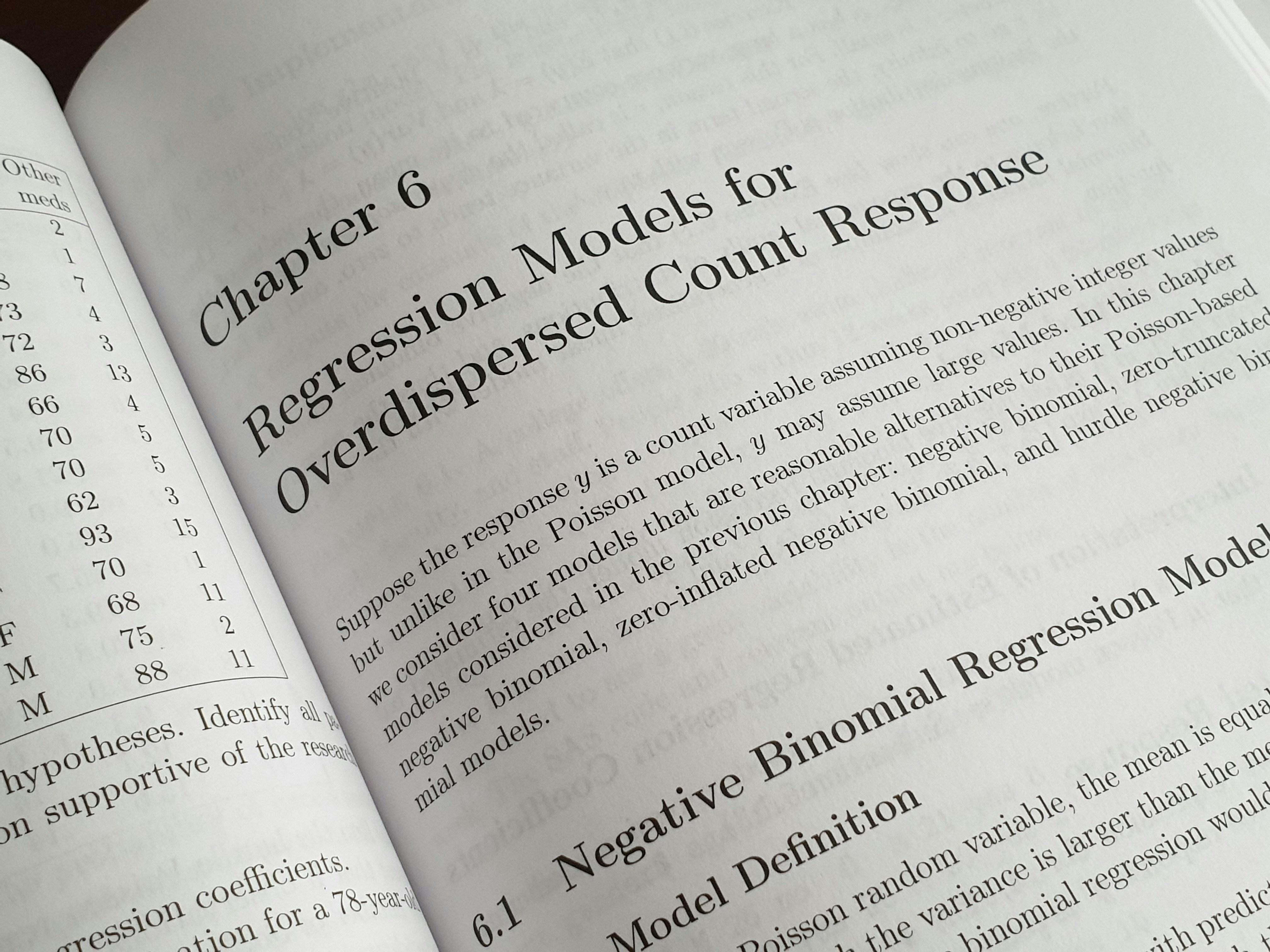Series

# Linear Regression

A gentle introduction to Linear Regression

• Bijon Setyawan Raya

• February 11, 2022

4 minsI am sure most of you reading this post right now are familiar with the following graph, and we did use $y = b + ax$ equation in high school or college to find the distance between two points in a 2D coordinate.

Not it is able to determine the distance between two points, but it can used to get a machine learning model to learn better.

Let's say that we have an Iris dataset from sklearn-learn. Plotting it will give us the following visualization.

Clearly, we can see that there are two features involved in the classification: sepal_length and petal_width.

The relationship between these two features can be written as follow.

$y = \beta_0 + \beta_1 \times x$

You should know that the intercept or $\beta_0$ is the starting point of the regression line. Whether the line is going up or down depends on the $\beta_1$ and the data. If $\beta_0 = 0$, it means that our regression line will start from $0$.

Expressing the equation like we did above is quite cryptic for people who don't have strong mathematical background. Since we are using the Iris Dataset, we can translate the equation into a more readable form.

$\text{petal width} = \beta_0 + \beta_1 \times \text{sepal length}$

From the translation above can tell us the relationship between those two variables. Now you will be wondering their correlations whether sepal_length and petal_width are correlated or inversely correlated.

sepal_length and petal_width are said to be correlated when sepal_length increases, the petal_length also increases. Conversely, sepal_length and petal_width are said to be inversely correlated when sepal_length increases, but the petal_width decreases.

With a regression line, it can help us to predict the $y$ value given a single $x$ value. However, most predictions made by the regression line are not always accurate since its ability to predict depends heavily on $\beta_0$ and $\beta_1$. If the values $\beta_0$ and $\beta_1$ are not tweaked correctly, the regression line will sit right far from most data points. Let's see an example down below where datapoints are far from the regression line.

When the regression line, which is the green line, sees $x = 0$ then it predicts $y = -3$. In reality, $y$ should be $0.5$ when $x = 0$. Meaning that our regression line is very bad at prediction. Since our regression line is bad at predicting, that indicates that we have a huge Mean Squared Erorr value.

Calculating the MSE for the graph above, we have

\begin{aligned} MSE &= \frac{47.59}{5} \\ &= 9.518 \end{aligned}

Mean Squared Error (MSE) has always been used to measure the quality of regression lines. If the MSE of a certain regression line is miniscule, we can say that the regression line is relatively better at prediction. If the MSE of a certain regression line is large, then it's the opposite. Here is the equation for MSE.

$MSE = \frac{1}{n} \sum_{i=1}^{n} (\hat{y}_i - y_i)^2$

where $n$ is the number of data points, $\hat{y}_i$ is the predicted value, and $y_i$ is the actual value.

Let's see another example where the data points are close to the regression line.

\begin{aligned} MSE &= \frac{1.74}{5} \\ &= 0.348 \end{aligned}